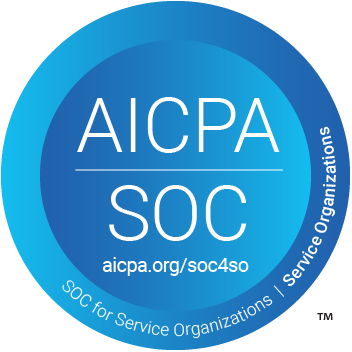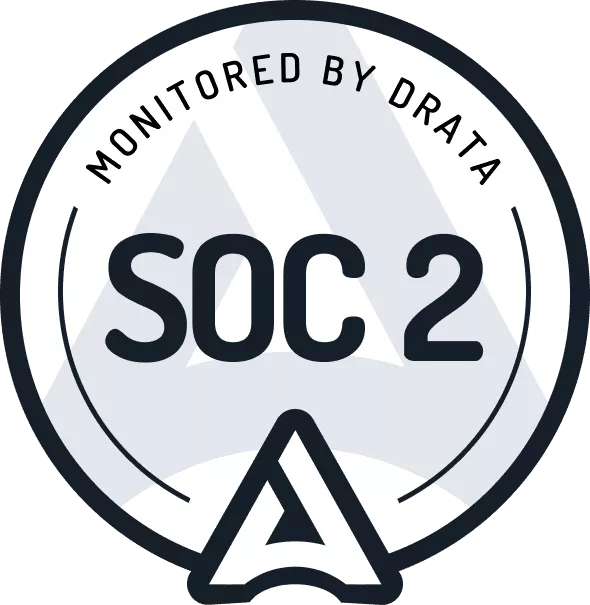# How to Calculate the Lease Liability and Right-of-Use Asset for an Operating Lease under ASC 842

## What Is an Operating Lease?

This post assumes some prior knowledge. If you're unaware of the new lease accounting standard, ASC 842, here's a quick summary

Akin to ASC 840, the new lease accounting standard ASC 842 prescribes the lessee to determine the lease classification. However, under ASC 842, it's no longer the classification between operating leases and capital leases. It's now operating leases and finance leases.

When transitioning to the new lease accounting standard, the standard allows you to grandfather previous lease classification under ASC 840. For more on this, refer here. This means the operating leases under ASC 840 can be carried forward as operating leases under ASC 842.

If it's a new lease under ASC 842 and you're unsure what the classification should be, we have extensive material in determining if the lease meets the definition of an operating or finance lease.

Under ASC 842, regardless of the lease classification, the lease is coming on the balance sheet. A lessee must recognize a right-of-use (ROU) asset and lease liability. The only exception is if the lease is less than twelve months long. Given this, accountants in your organization will need to know how to calculate an operating lease in compliance with ASC 842.

### Accounting for an Operating Lease Under ASC 840

Previously, accounting for operating leases under ASC 840 was relatively straight forward. Under ASC 840-20-25-1, it prescribed the following treatment:

Rent shall be charged to expense by the lessee over the lease term as it becomes payable. If rental payments are not made on a straight-line basis, rental expense nevertheless shall be recognized on a straight-line basis unless another systematic and rational basis is more representative of the time pattern in which use benefit is derived from the leased property, in which case that basis shall be used.

From the perspective of accounting simplicity, the preferred classification was an operating lease under ASC 840.

### Accounting for an Operating Lease Under ASC 842

ASC 842 requires lessees to bring all leases on the balance sheet. The only exception is if at lease commencement, the lease term of 12 months or less.

So what does this mean? It's essentially like accounting for all your leases as if they were capital leases under ASC 840. Under ASC 842, an operating lease you now recognize:

• A lease liability: the present value of all known future lease payments
• Right of use asset: the lessee's right to use the leased asset. Which is amortized over the useful life of the asset.

So, where to begin? If you're unsure, refer to our initial recognition guidance. This covers the fundamentals inputs of recognizing a lease liability and then a right of use asset

### Modification Accounting for an Operating Lease Under ASC 842

The devil is in the detail when calculating modifications. If you’re unsure of what a lease modification is, refer to detailed guidance here

When a modification occurs, the lease calculation must be updated to reflect the changes in contractual details. The two inputs that can be changed are:

• Lease term
• Lease payments

Either or both can change depending on the modification. When a lease modification occurs, you must update the present value calculation to reflect the updated lease liability value as the future payments have been changed.

Example 2 illustrates how to calculate the lease liability and right of use asset when a lease modification occurs.

## Practical Examples

So you’ve read the guidance, and now you’re good to go. You have a basic understanding that the lease liability is the present value of the future lease payments at commencement. The right of use asset builds off that value, with a few other potential nuances throw-in.

So it’s time to put theory into practice and calculate the lease liability. We’ll walk through step by step of a basic lease example. If you would like a copy of the lease calculation in excel, please reach out to [email protected]. Obtaining the excel file will also allow you to use it as a template for future lease calculations.

Lastly, some areas to note in relation to the calculation methodology:

• Calculations will be done daily: that’s right, interest and amortization are calculated for each individual day in the lease. This gives the ultimate accuracy of numbers when modification accounting occurs. Furthermore, the time to set up a daily calculation compared to a monthly calculation is negligible given it’s the same formulas used in each row.
• XNPV present value formula: The XNPV formula will be used in Microsoft Excel. This takes into consideration the date of payments and therefore gives a more accurate present value amount.

If you’re unsure of the basic principles of present valuing or unsure what formula you should use in Microsoft Excel, refer to our article on How to Calculate the Present Value of Future Lease Payments.

## Example 1 Scenario

• Start date: 2020-1-1
• Accounting End date: 2020-12-31
• Fixed Payment Amount: \$10,000
• Payment Frequency: Monthly
• Lease classification: Operating Lease
• Discount rate: 7.00%

### Step 1 - Work Out Future Lease Payments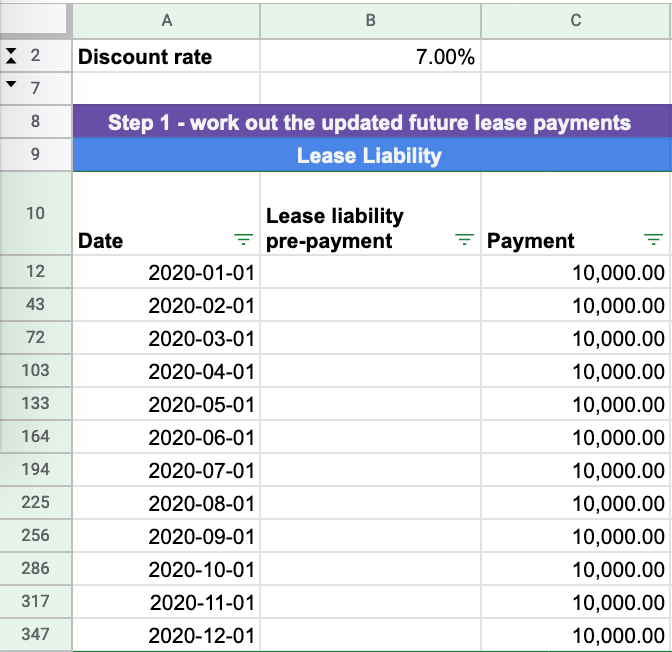The lease liability is the foundation of lease accounting under ASC 842, as the lease liability is the present value of future lease payments. So the first input of the calculation to figure out is what are future known lease payments at commencement. These are the figures we are going to use to establish the present value

Refer here for explicit guidance of what should be included in the lease payments. In reference to Example 1, it is 12 payments of \$10,000 falling on the first of each month for the period between 2020-1-1 to 2020-12-31.

### Step 2 - Determine the Discount Rate and Calculate the Lease Liability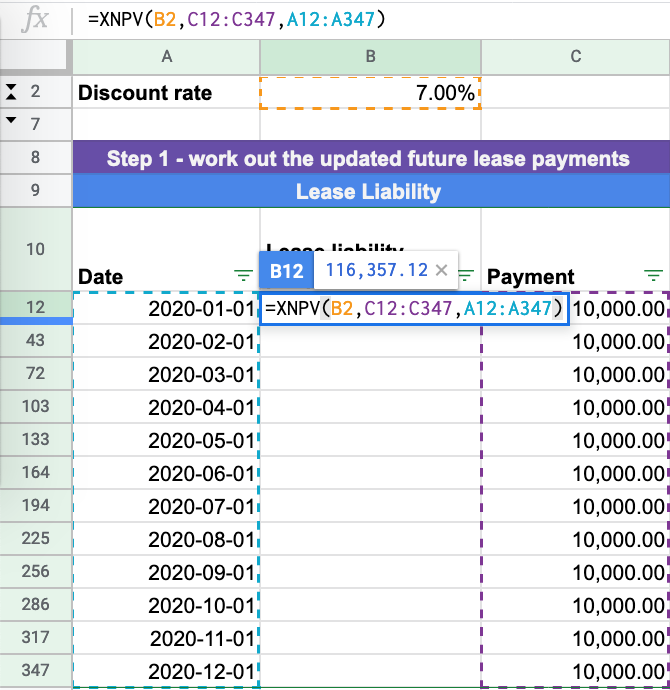From an accounting judgement perspective, this is one of the more complex areas of lease accounting. For further information on how to determine the appropriate discount rate refer to this material

Once you have determined the discount rate, you have all the inputs to complete the XNPV formula in Microsoft Excel. In reference to Example 1, the discount rate is 7%. As a result, in the XNPV formula you will input:

• Rate: 7%
• Values: \$10,000 at the start of each month
• Dates: 2020-1-1 to 2020-12-31

Inputting this data will give you the present value of the future lease payments of \$116,357.12. This is the lease liability amount at initial recognition.

### Step 3 - Calculate the Right-of-Use Asset Value

The lease liability is the foundation of the right of use asset. When calculating the right of use asset value, it can consist of several inputs. For further discussion of those inputs, refer here

In the above example, it’s straightforward, the right of use-value equals the lease liability value of \$116,357.12

### Step 4 - Calculate the Unwinding of the Lease Liability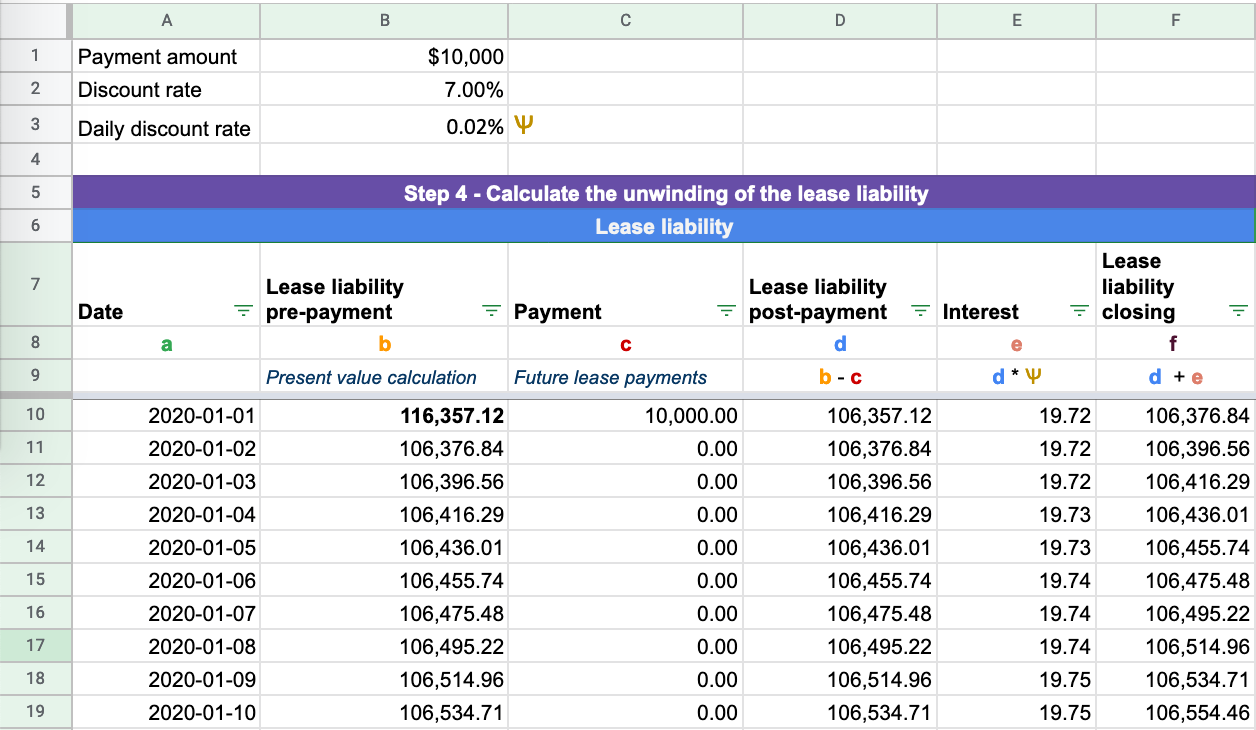You now have your opening balance for the lease liability and ROU asset calculated in compliance with ASC 842. The next step is to calculate the unwinding of the lease liability to \$0. We already have all the necessary information given there have been no modifications to the lease term.

We’ll break down the calculation in reference to the above picture column by column. The formulas used do not change row to row:

Column A - Date - Captures each day within the agreement from 2020-1-1 to 2020-12-31: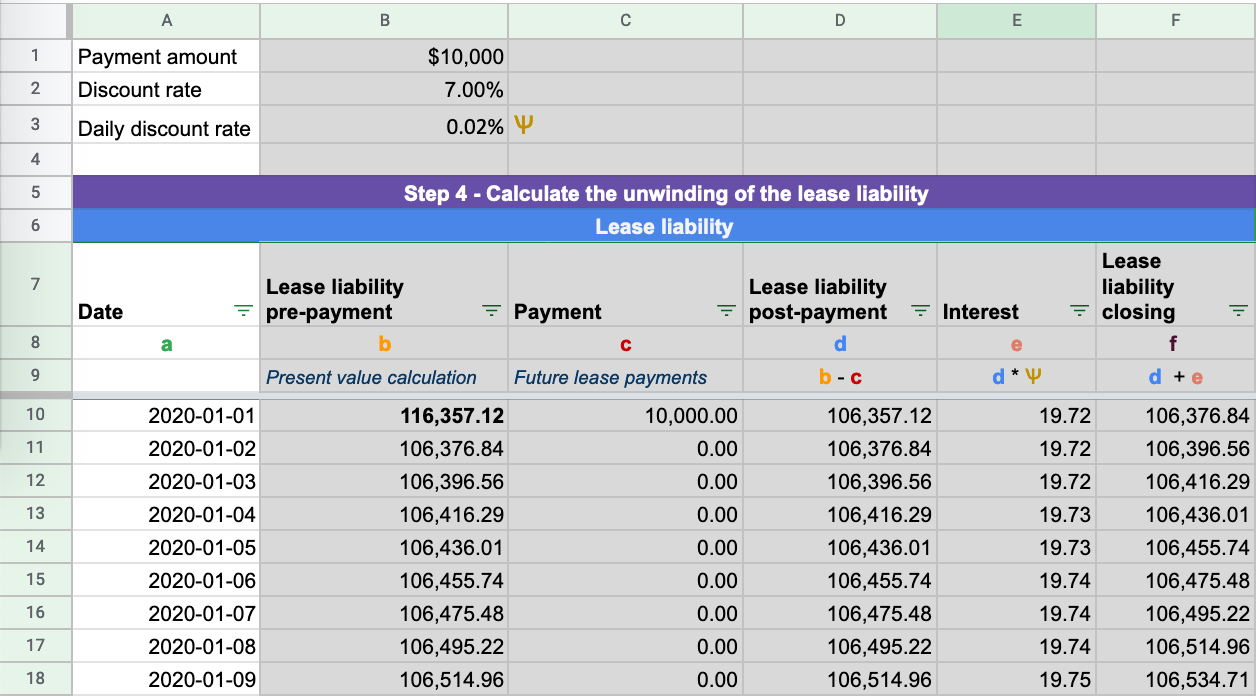Column B -Lease liability prepayment- Where the present value XNPV formula is input for each row: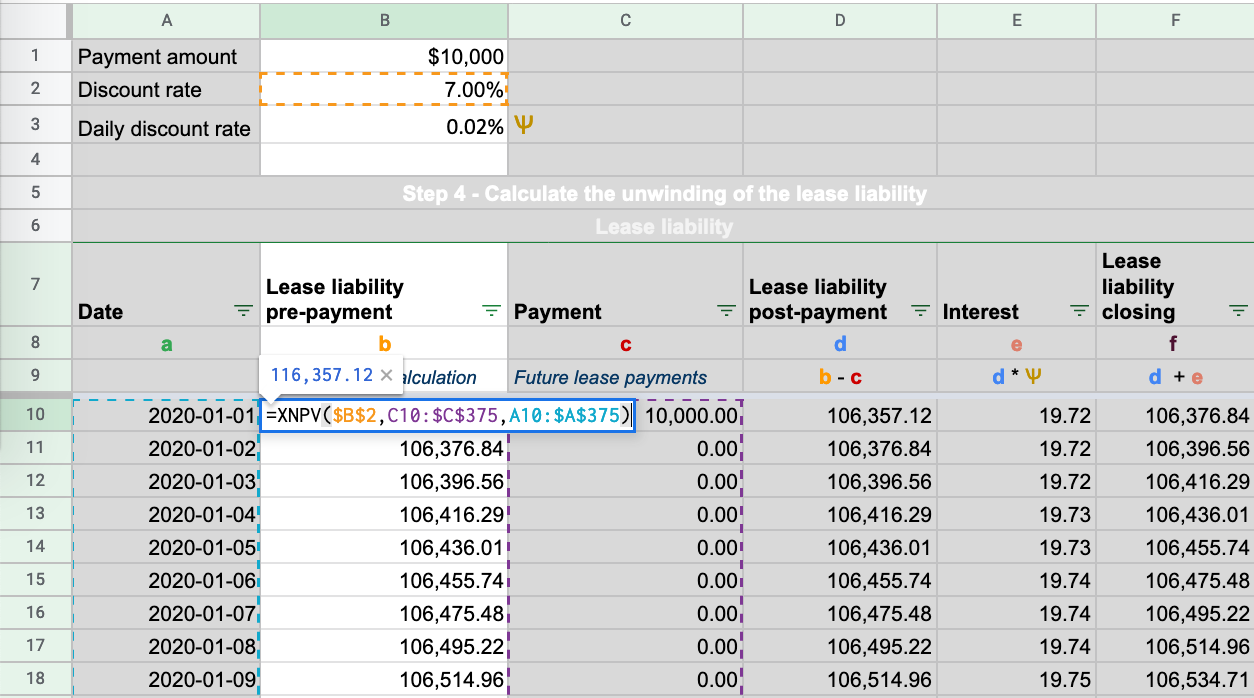Column C - Payment - Future lease payments at each particular date: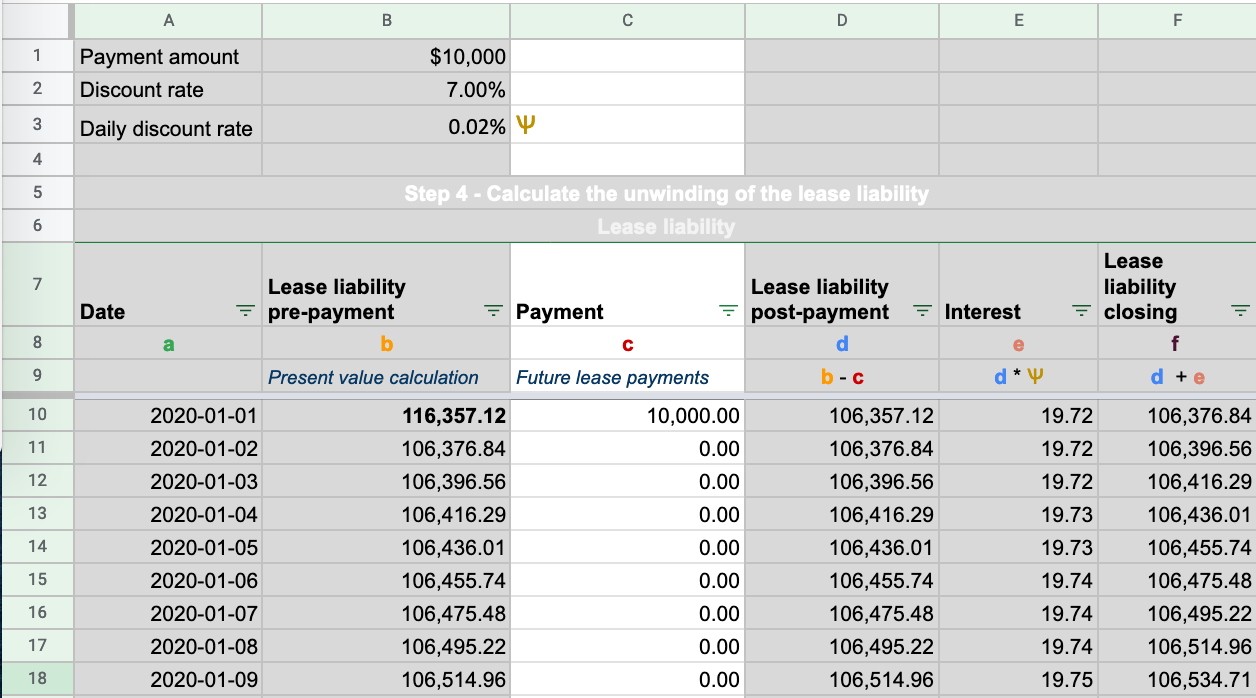Column D - Lease liability post-payment - This is the lease liability amount post-payment. Payments reduce the lease liability balance: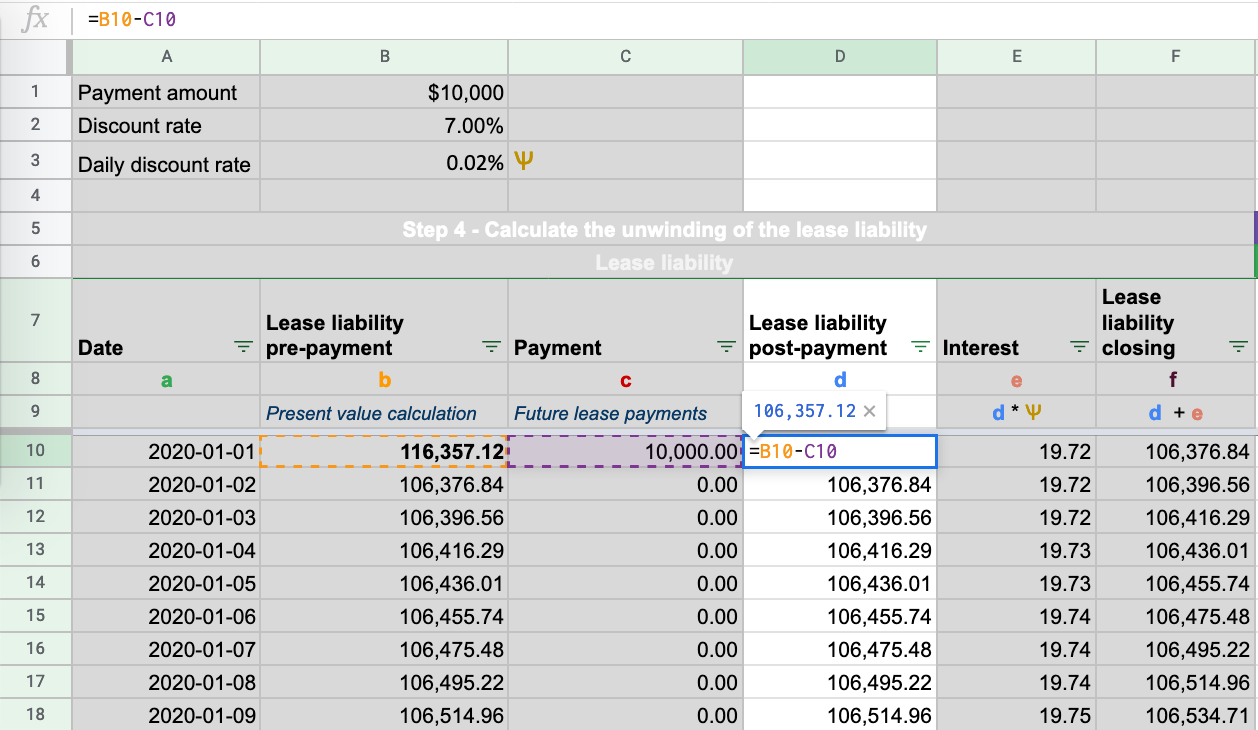Column E -Interest- This is the daily interest amount calculated on the lease liability based on the daily discount rate: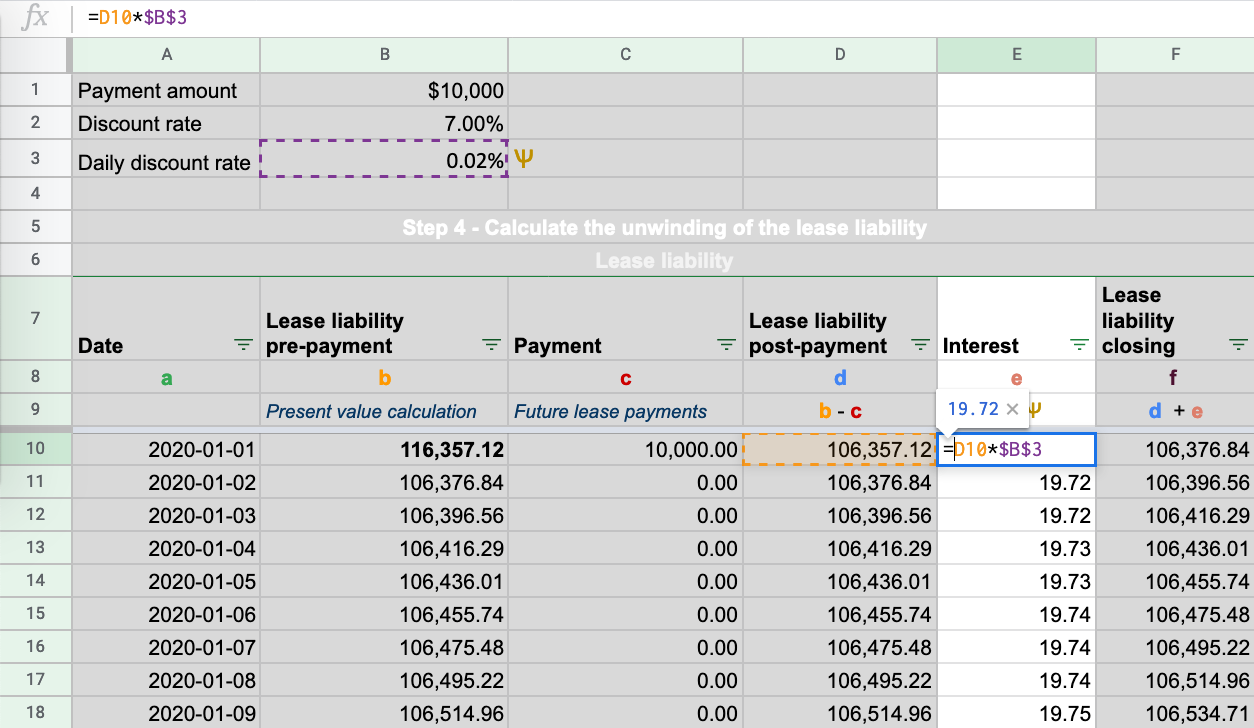To calculate the daily discount rate is the following:  (1+discount rate)^(1/365)-1

Column F - Lease liability closing - the lease liability post-payment plus the interest. It is the closing balance of the lease liability. This balance is brought forward to the next day: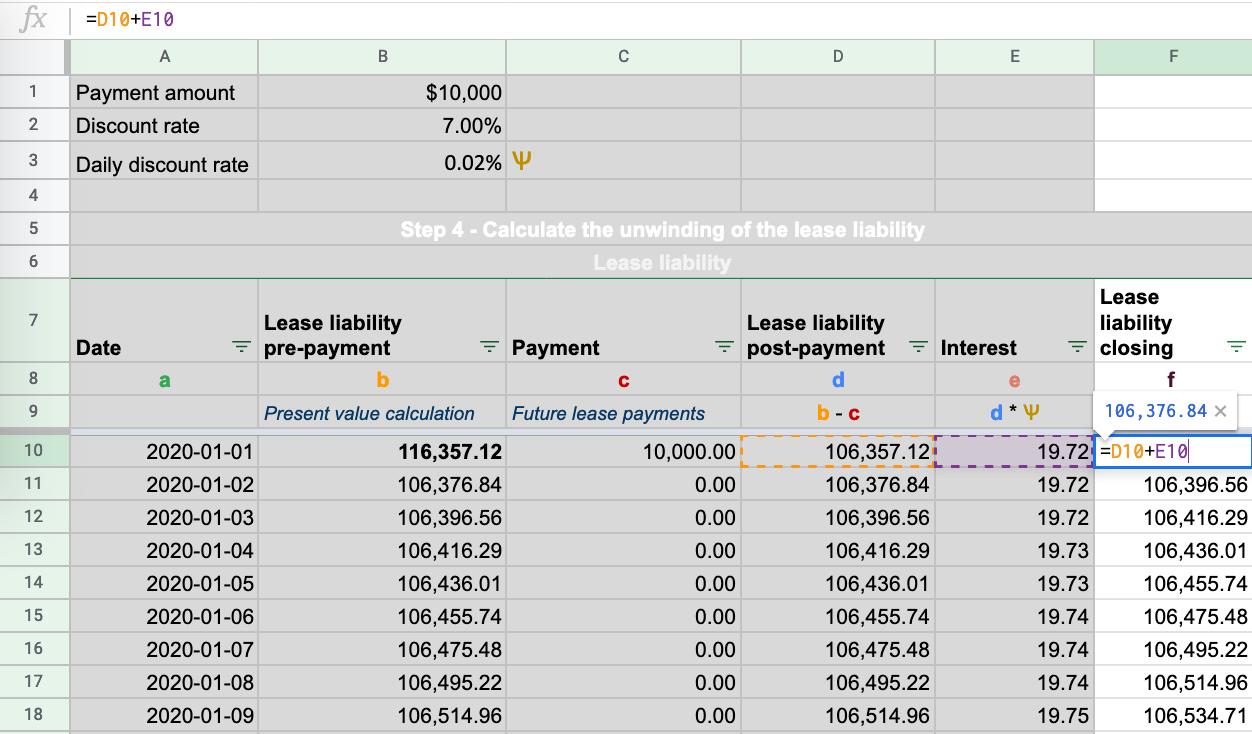To ensure your lease liability has been calculated correctly ensure it unwinds to zero as shown in the below animation: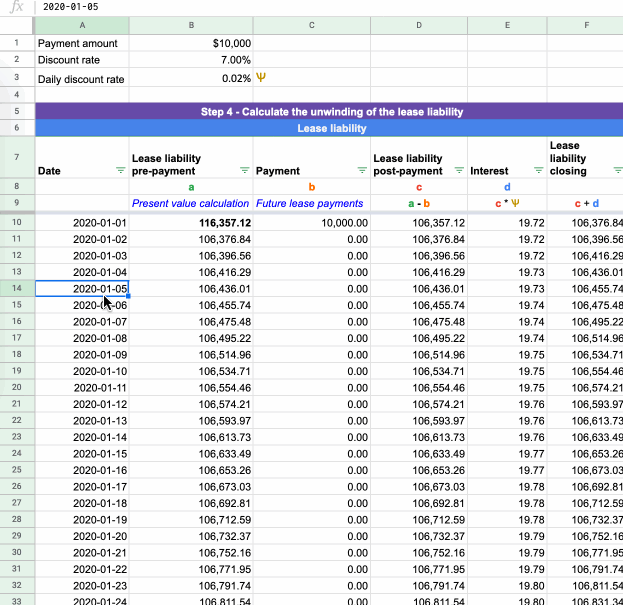### Step 5 - Calculate the Right-of-Use Asset Amortization Rate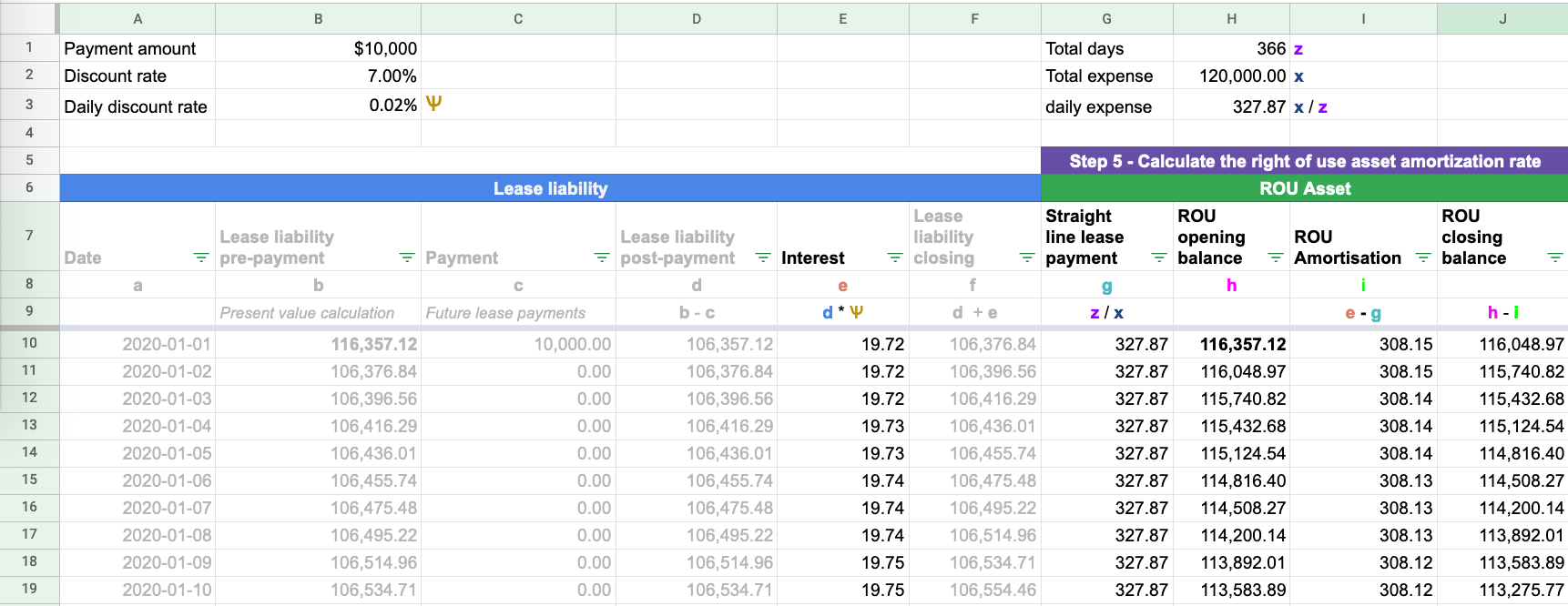You have calculated the unwinding of the lease liability and have the ROU asset opening value. Here are the following steps to take when calculating the ROU asset amortization schedule:

a) Calculate the straight-line lease payment: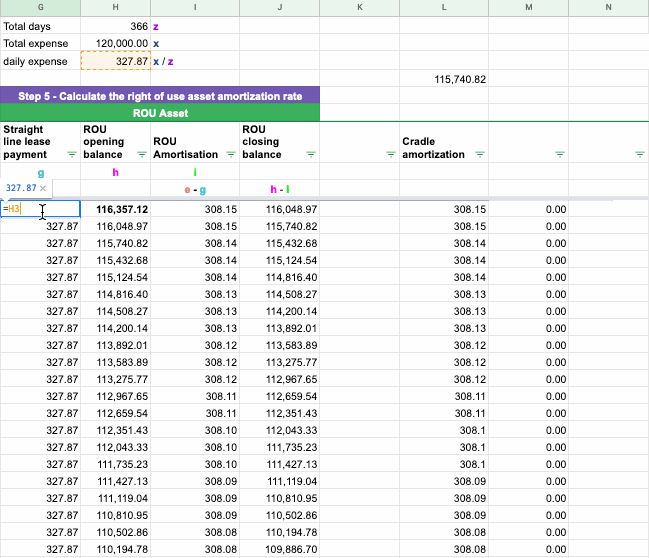Add up the total lease expense that is known at the outset of the commencement of the lease. The total lease expense is net of items like direct costs and lease incentives starting before the commencement.

Using Example 1 the total lease expense is \$120,000 (\$10,000*12).

Area to note: If there is an amount that is added to the ROU asset, for example, direct costs, this amount will also be added to the straight-line lease payment amount.

b) Calculate the number of days in the agreement: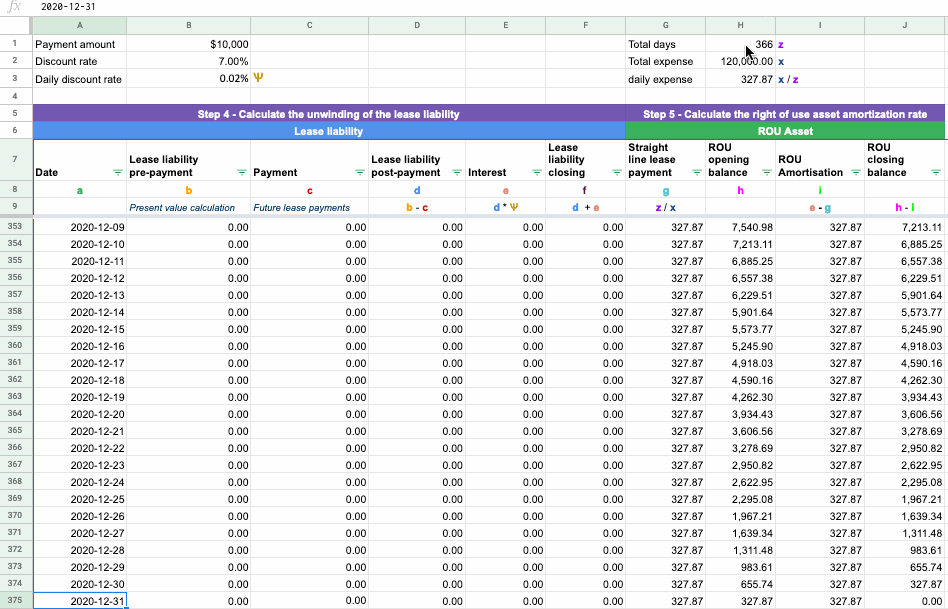A quick way to do this in Excel is to use the COUNT function and count the number of rows used (it can be any column). Using Example 1 the total number of days in the lease is 366 days (2020 is a leap year).

c) Divide total expense by number of days to get the straight-line lease expense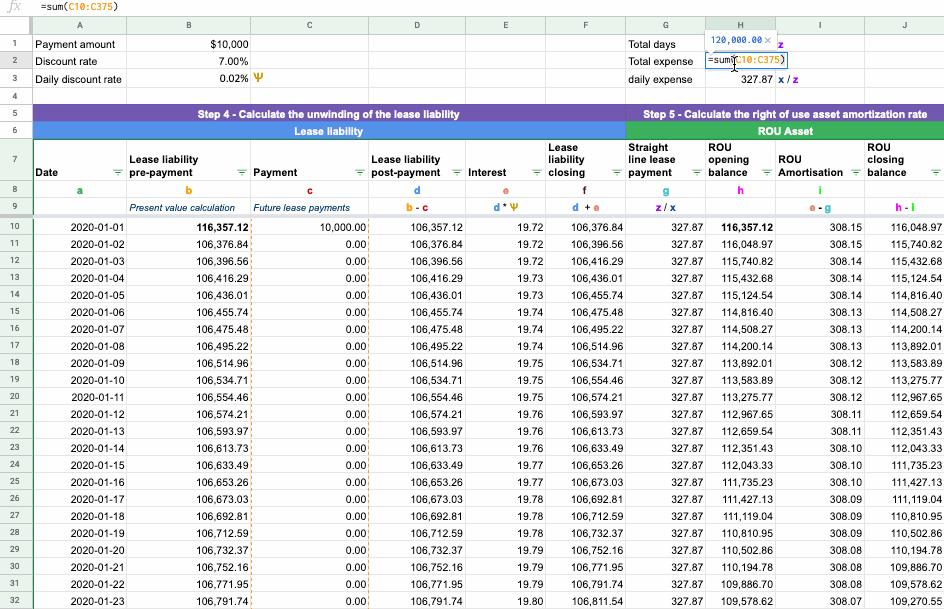Using Example 1 the straight-line lease expense is \$327.87 (120,000 / 366)

d) Subtract the daily straight-line lease expense by the daily interest expense which gives you the amortization expense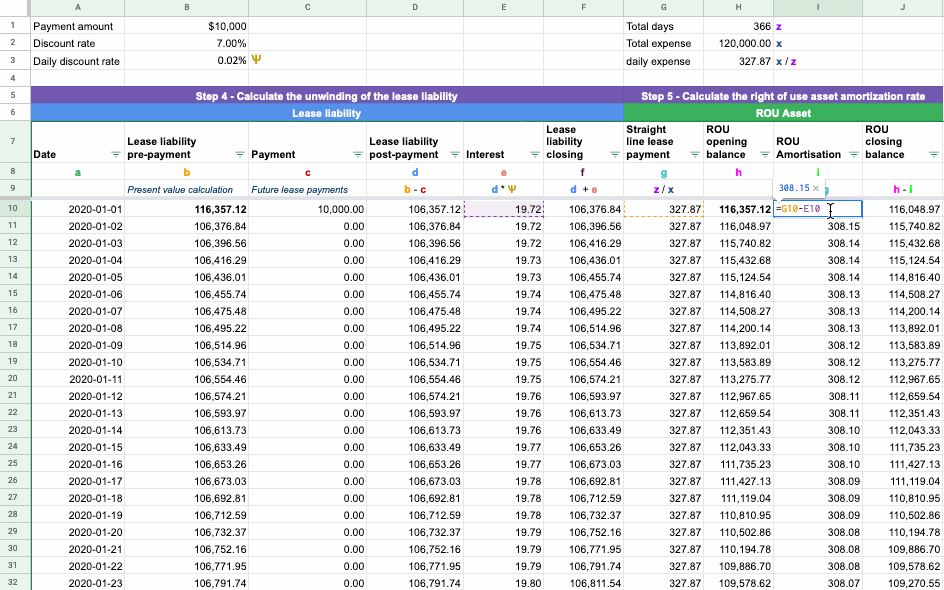Using Example 1 on 2020-1-1 the ROU amortization expense is \$308.15 (\$327.87 - 19.72)

e) Ensure the ROU asset is amortized to \$0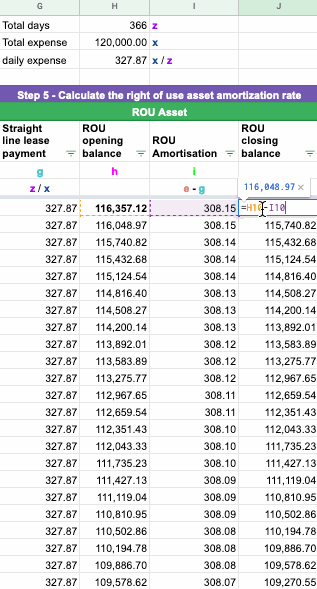Assuming there are no changes you have now completed the calculation for an operating lease in adherence to ASC 842.

## Example 2 Scenario - Modification Accounting

Example 2 will cover how the calculations work for a particular modification to an operating lease terms accounted under ASC 842. If you want more information on what prompts modification accounting and the different types of lease modifications, refer here

We’ll now go through the following calculation steps of a modification that increases the fixed payments for an operating lease under ASC 842.

Using the details of Example 1:

• Start date: 2020-1-1
• Accounting End date: 2020-12-31
• Fixed Payment Amount: \$10,000
• Payment Frequency: Monthly
• Lease classification: Operating Lease
• Discount rate: 7.00%
• Direct costs incurred: 25,000

Modification to the terms:

• On 2020-10-16, the fixed payment amount increased to \$12,000 on 2020-11-1
• The appropriate discount rate at modification is 6.00%

The key differences compared to Example 1 are:

• Direct costs of \$25,000 at initial recognition: This will increase the ROU asset to 141,357.12 (\$116,357.12 + \$25,000) and the straight line lease payments to 396.17 (\$25,000 + 120,000)/366.
• A modification of the lease agreement occurs on 2020-10-16 for fixed payments to increase to \$12,000 starting on 2020-11-1

If you would like the excel calculation for Example 2, please reach out to [email protected]

### Step 1 - Work Out the Modified Future Lease Payments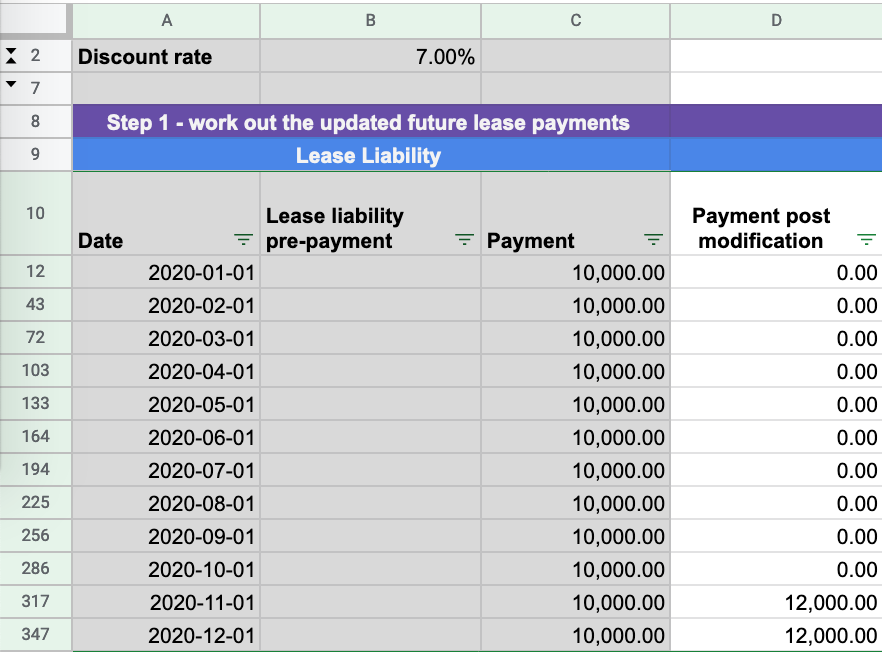To calculate the present value of the modified future lease payments, an additional column has been added. If you do not add a column, it will impact all previous calculations as you’re changing the future cash flows used to present value the lease liability. In reference to Example 2, on 2020-10-16 the future lease payments on 2020-11-1 and 2020-12-1 have been modified from \$10,000 to \$12,000.

### Step 2 - Determine the Appropriate Discount Rate and Re-calculate the Lease Liability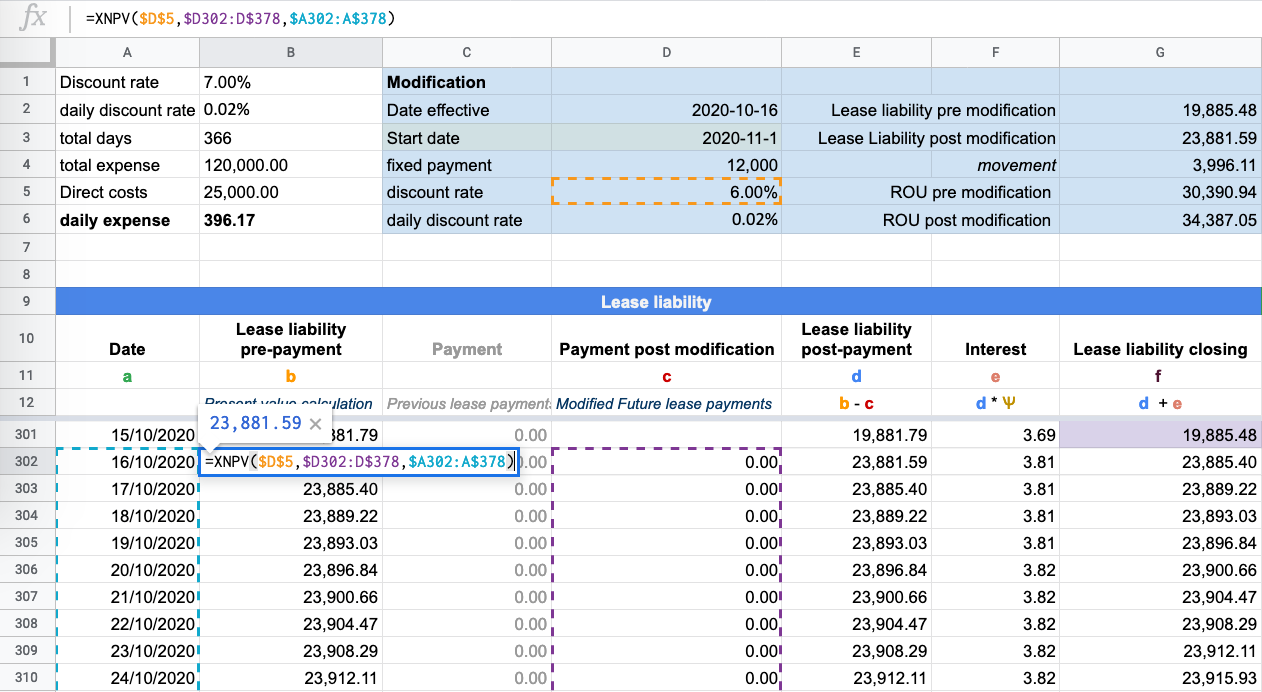In relation to Example 2, the following have changed in relation to the calculation on 2020-10-16:

• An updated discount rate of 6% in CELL D5
• Values: The XNPV formula values will present value the updated future payments of Column D.

Based on adding a new column the following updates will need to be made to the calculation:

a) Lease liability post payment will subtract payments from column D as opposed to column C: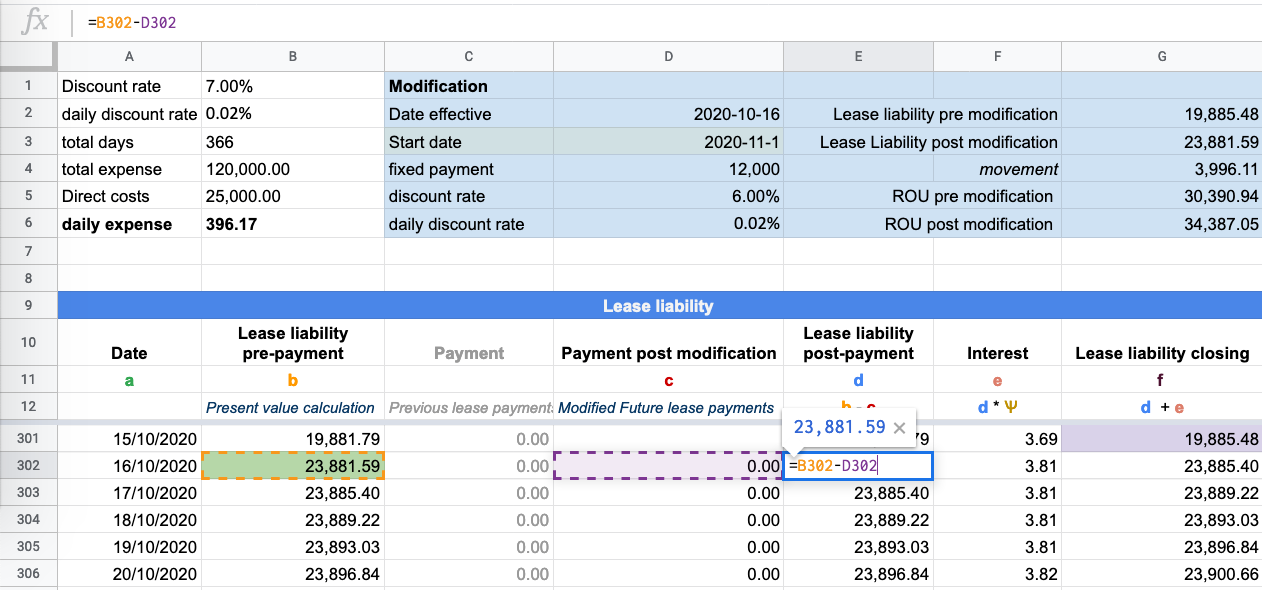b) Daily interest calculation will use the updated daily discount rate: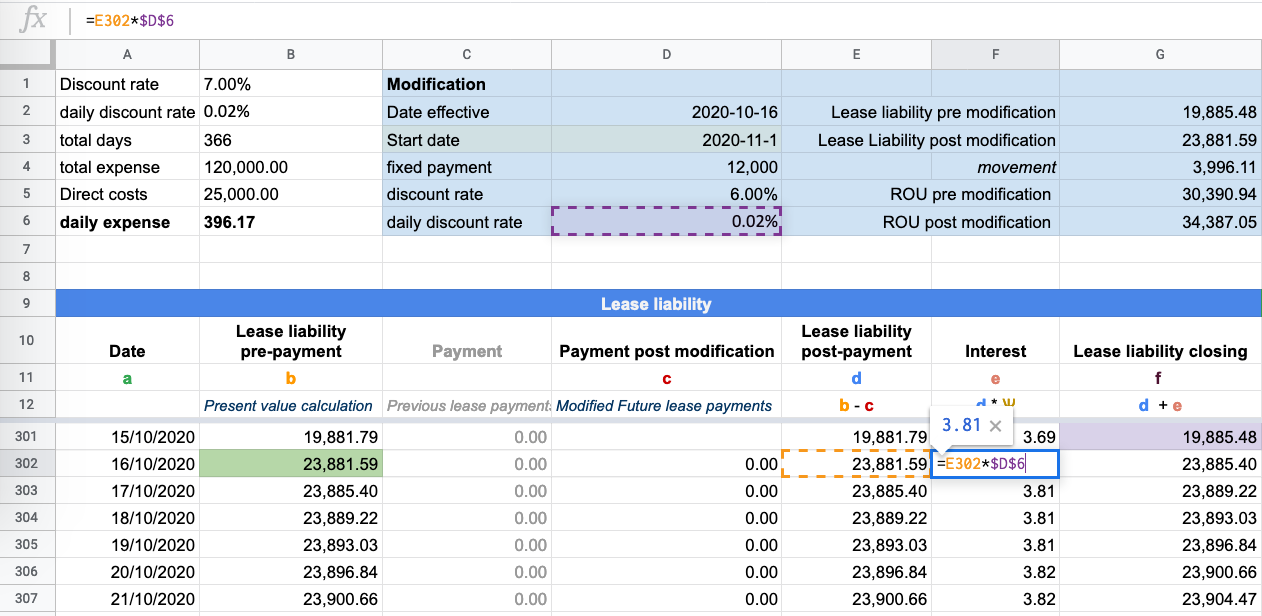In Example 2, the discount rate has increased from 6% to 7%. As a result, the daily discount rate for calculating the interest on the lease liability needs to be updated.

Once you have determined the discount rate, you have all the inputs to complete the updated lease liability's present value calculation based on the modified terms.

As a check, ensure the lease liability post modification unwinds to \$0 based on the updated lease inputs.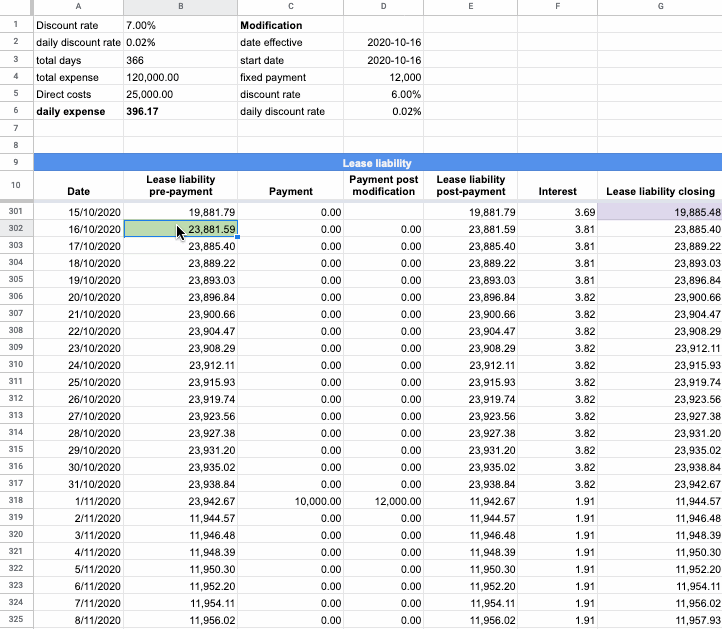If you’ve forgotten to make the correct updates to the calculation the lease liability will not unwind to zero by 2020-12-1.

### Step 3 - Capture the Modification Movement and Apply That to the ROU Asset Value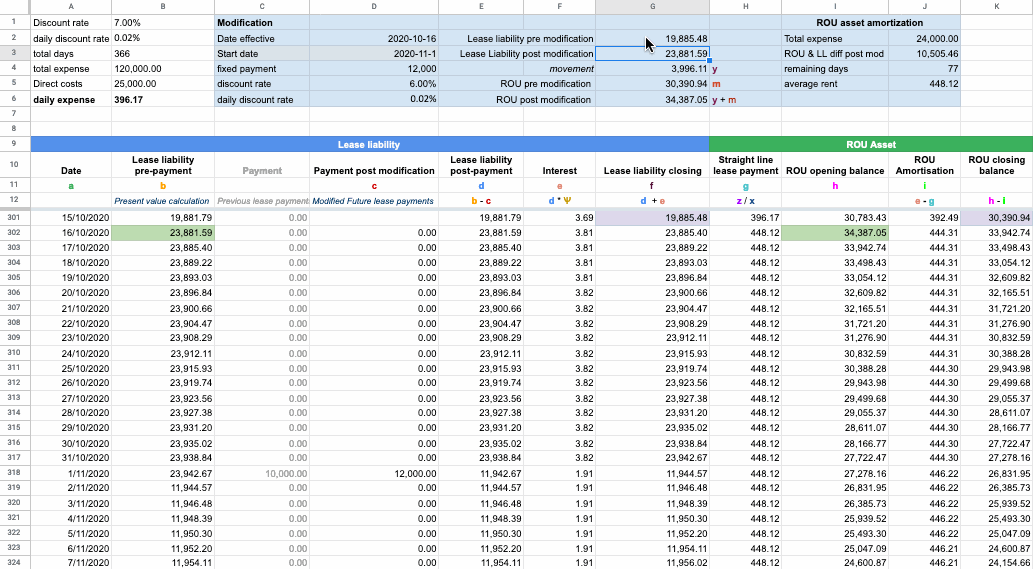The closing balance of the lease liability before the modification on 2020-10-15 is \$19,885.48. The lease liability value post modification based on the updated future cash flows is \$23,881.59, which is an increase of \$3,996.11 to the lease liability value. This amount needs to be added to the ROU Asset as that’s the other side of the remeasurement journal entry which is:

Dr ROU Asset \$3,996.11

Cr Lease liability \$3,996.11

As a result, the ROU asset balance before modification on 2020-10-15 was \$30,390.94. Post-modification, the ROU asset balance is \$34,387.05.

### Step 4 - Update the ROU Amortization Rate

When there is a change to the contractual terms, it results in a remeasurement of the lease liability, which then the ROU Asset value is impacted. As a result, the amortization rate must change to ensure the ROU asset is amortized to zero. This is one of the trickier areas of accounting for an operating lease under ASC 842.

To ensure the balance is correctly amortized to \$0 you must:

a) Calculate the total remaining lease expense: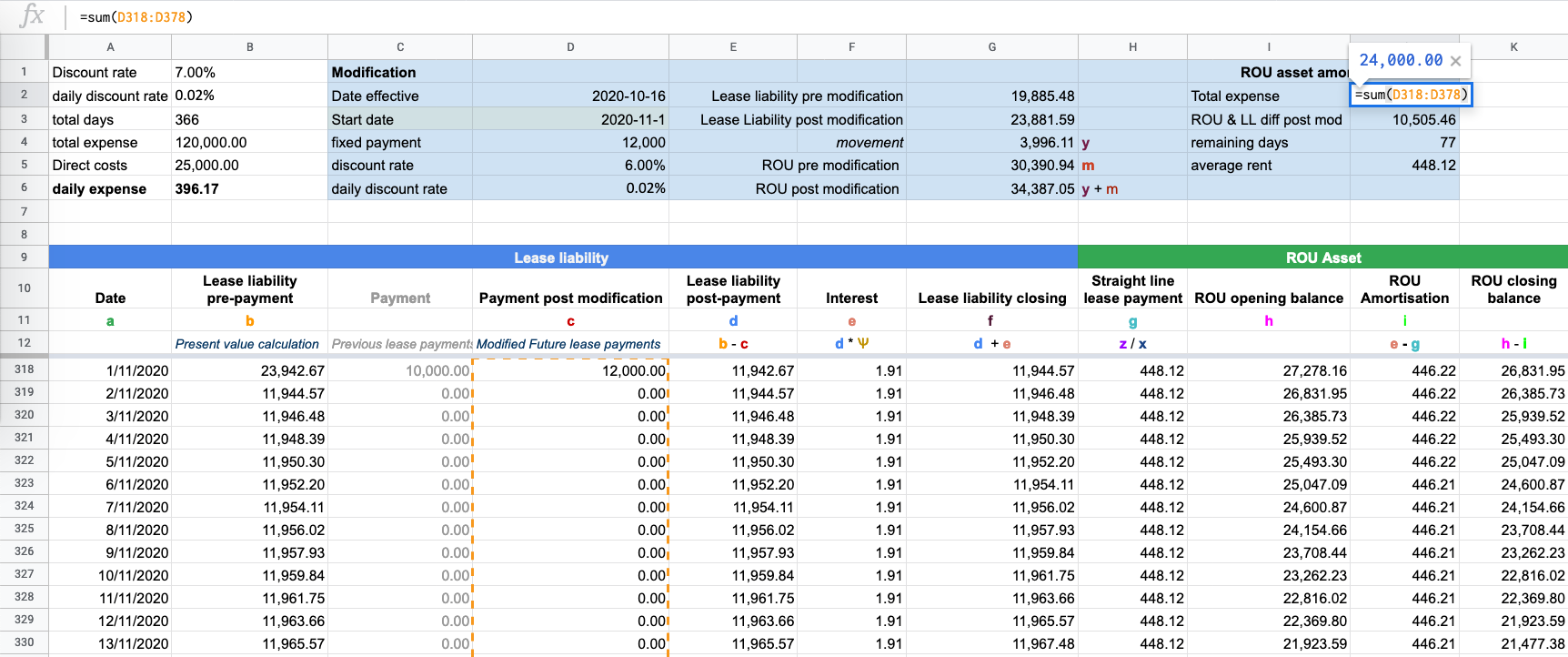In Example 2 the total lease expense is \$24,000 with two lease payments occurring on 2020-11-1 & 2020-12-1.

b) Calculate the difference between the ROU asset and the lease liability post-modification: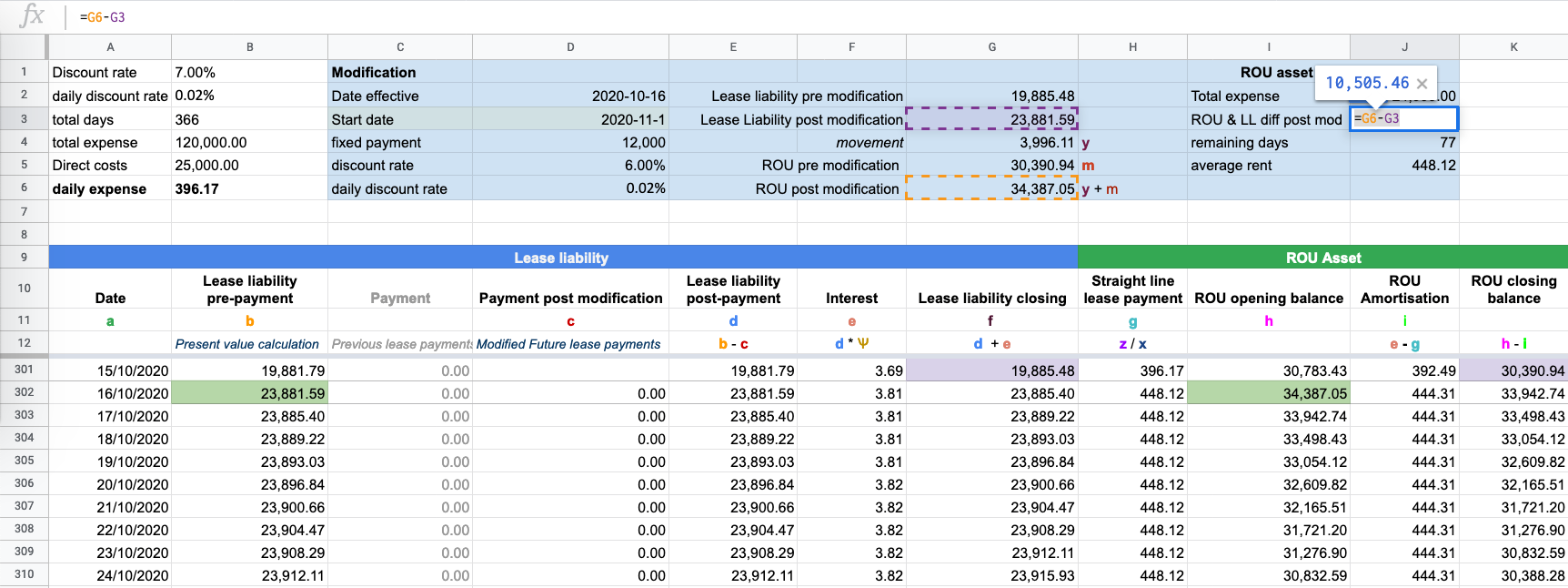In reference to Example 2, the ROU asset post-modification is \$34,387.05 while the lease liability is \$23,881.59 resulting in a difference of \$10,505.46

c) Calculate the number of remaining days of the useful life of the asset: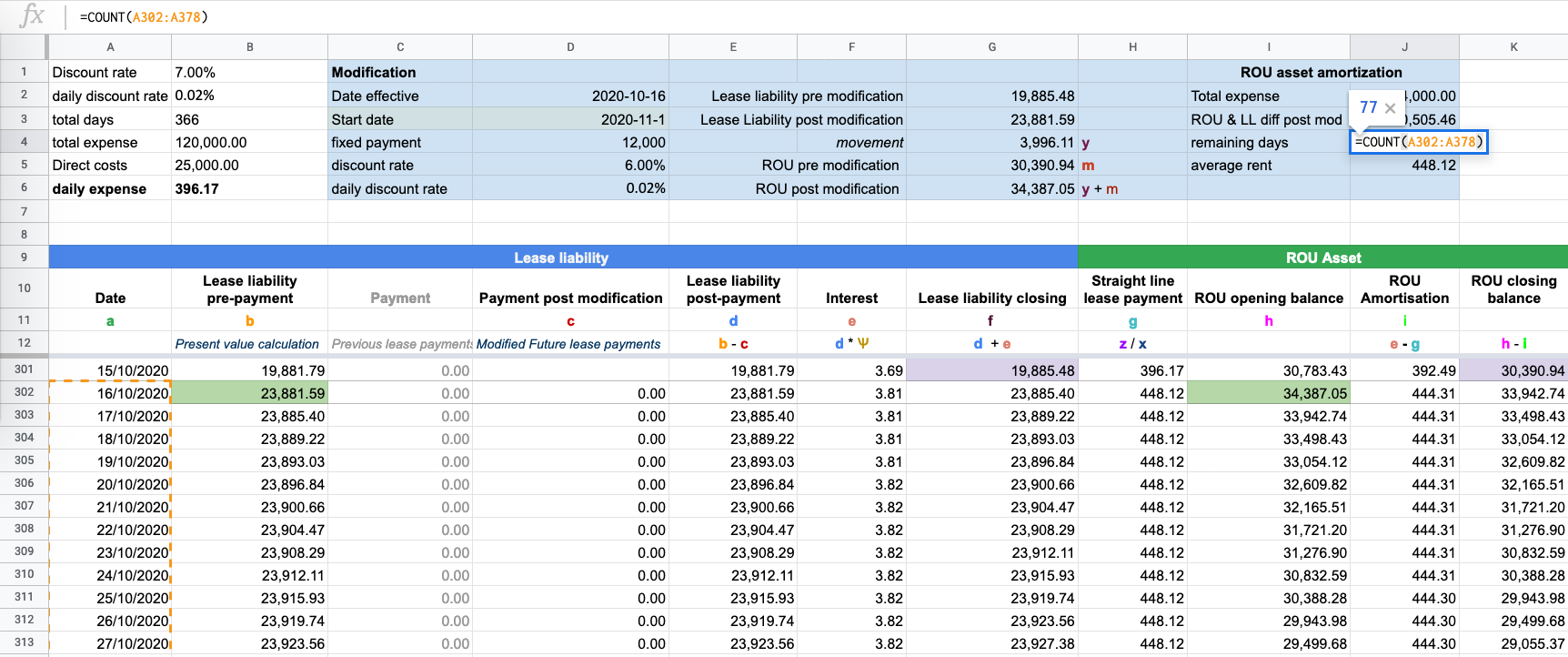In Example 2, it is 77 days which is calculated from the date of modification 2020-10-16 to the accounting end date 2020-12-31.

From those inputs you can calculate the average rent expense for the remainder of the lease:

• Total expense: \$24,000
• Difference between ROU and lease liability post modification: \$10,505.46
• Remaining days: 77

Straight line lease payment: \$448.12 (24,000 + 10,505.46) / 77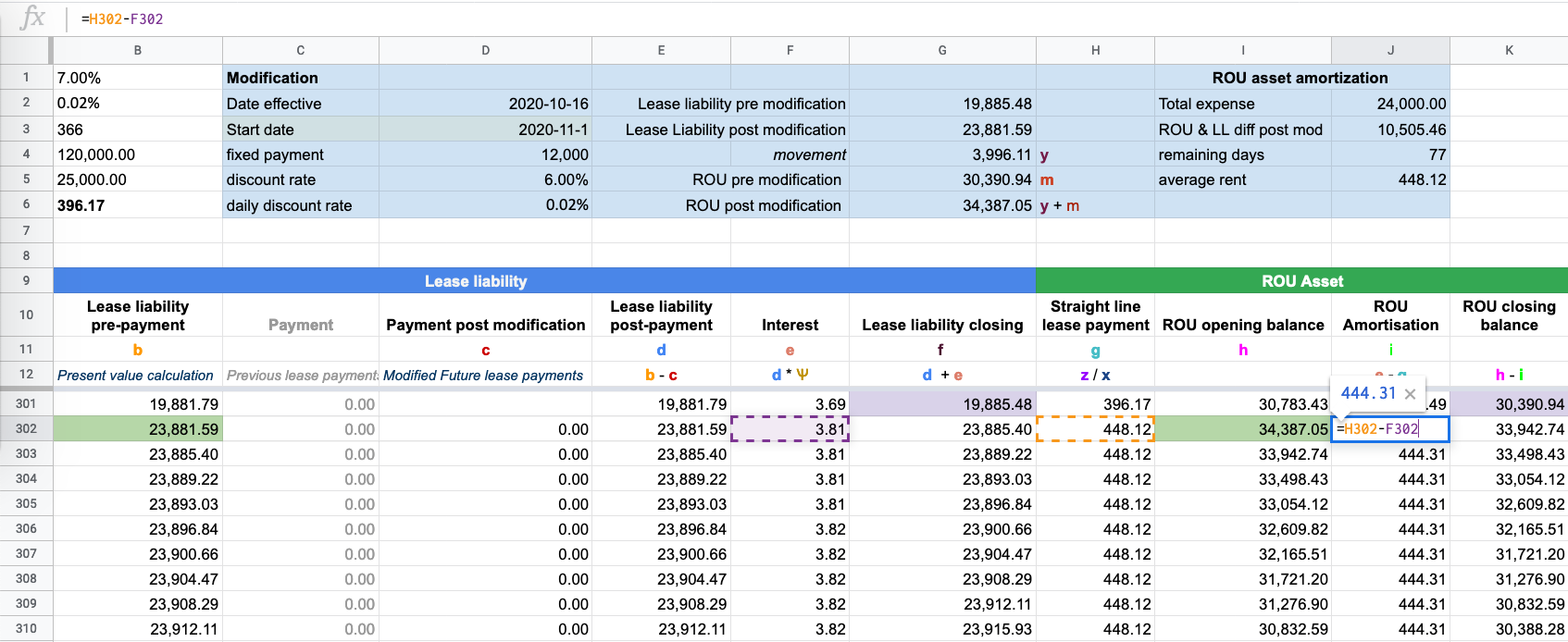Subtract the \$448.12 from the daily interest amount to get the ROU amortization amount. The amount of amortization incurred on 2020-10-16 was \$444.31. To ensure the calculation is correct, the ROU asset should be amortized to \$0 on 2020-12-31.

Once those steps are complete you have finished the calculations necessary for a modification of an increase in fixed payments for an operating lease under ASC 842.

## What About the Journal Entries?

If you're curious as to what are the journal entries for an operating lease under ASC 842 refer to the article The Journal entries for an operating lease under ASC 842.

## Try Cradle free for 30 days.

Starting at only \$99/month. Automate your lease accounting calculations, journal entries, and financial reports by simply entering the contractual details of the lease.

Start Free Trial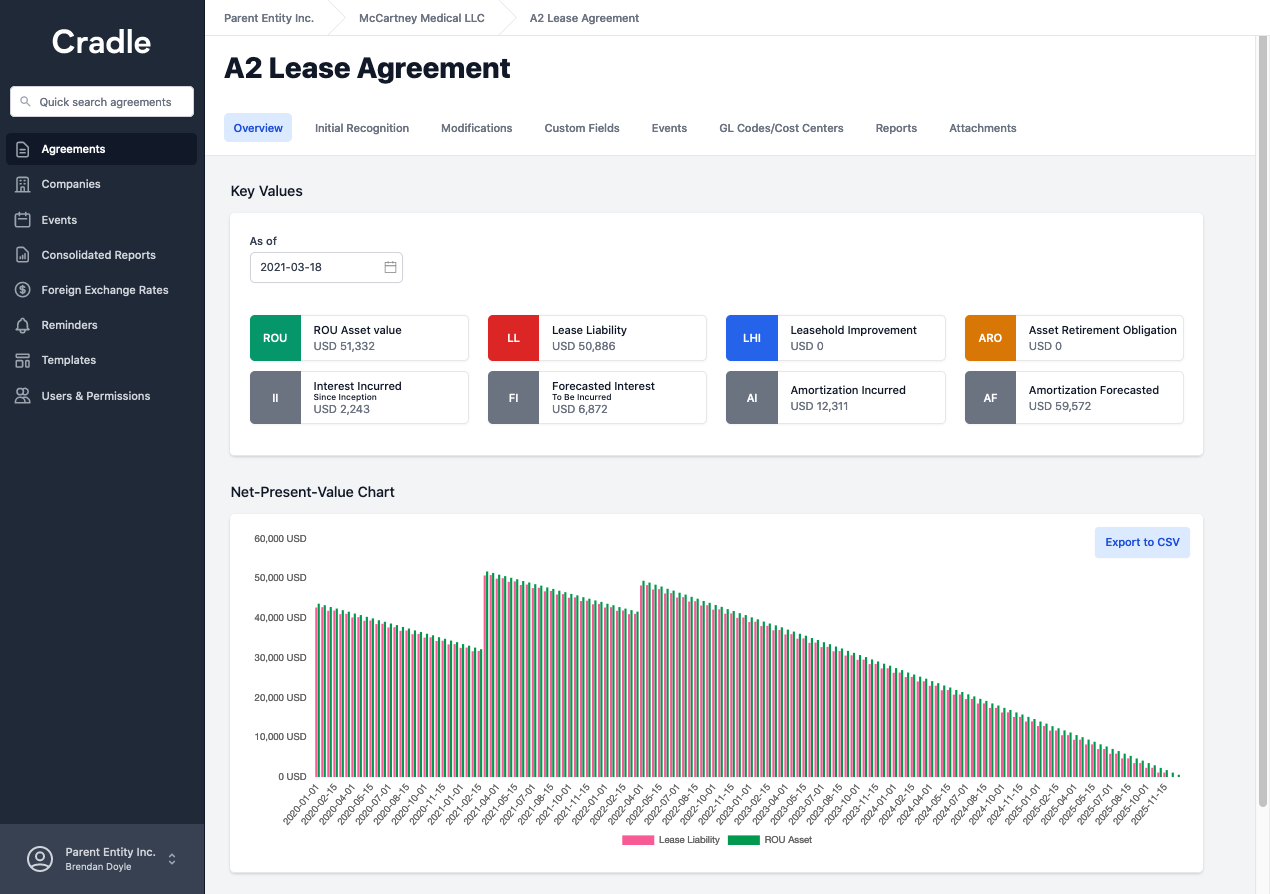## FooterHere at Cradle, our mission is simple; it's at the foundation of everything that we do. We want to make accountants' lives easier by leveraging technology to free up their time to focus on running the business.

140 Yonge St.
Suite 200
Toronto, ON M5C 1X6

US
Suite #73591
Torrance, CA 90503
USA

### Legal